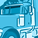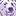# New to Qlik Sense

If you’re new to Qlik Sense, start with this Discussion Board and get up-to-speed quickly.

Announcements
Become an analytics expert with Qlik's new 15 week course: Applied Data Analytics using Qlik Sense. READ MORE
cancel
Showing results for
Did you mean:Creator II

## Dynamic sort Pivot table

I have created a variable for vSort for dynamic Sort Pivot table.

In the variable extension, I use the below formula but when I hit the related button, there is no sorting. I wonder if it is because my formula is wrong.  Hope I could get some guidance.  Many thanks as always.

='fabs(Pick(Match(Movement_Type,"TS1","TS2","TS3","TS4"),sum([S1_GCV_Mov_ HKD000]),sum([S2_GCV_Mov_ HKD000]), sum([S3_GCV_Mov_ HKD000]), sum([POCI_GCV_Mov_ HKD000]))~GCV_Mov|fabs(Pick(Match(Movement_Type,"TS1","TS2","TS3","TS4"),sum([S1_ECL_ Allw_ HKD000]),sum([S2_ECL_ Allw_ HKD000]), sum([S3_ECL_ Allw_ HKD000]), sum([POCI_ECL_ Allw_ HKD000])))~ECL_Mov'

Labels (1)
• ### Other

4 RepliesCreator II

Try the below expression:

fabs(Pick(Match(Movement_Type,'TS1','TS2','TS3','TS4')
,sum([S1_GCV_Mov_ HKD000])
,sum([S2_GCV_Mov_ HKD000])
, sum([S3_GCV_Mov_ HKD000])
, sum([POCI_GCV_Mov_ HKD000]))~GCV_Mov|
fabs(Pick(Match(Movement_Type,'TS1','TS2','TS3','TS4')
,sum([S1_ECL_ Allw_ HKD000]),sum([S2_ECL_ Allw_ HKD000])
, sum([S3_ECL_ Allw_ HKD000]), sum([POCI_ECL_ Allw_ HKD000])))~ECL_MovCreator II
Author

Thank you very much.

Sorry I have error when removing the single quote "Error in expression.  Garbage after expression '~'.Creator II

use this:

'fabs(Pick(Match(Movement_Type,chr(39)&TS1&chr(39),chr(39)&TS2&chr(39),chr(39)&TS3&chr(39),chr(39)&TS4&chr(39))
,sum([S1_GCV_Mov_ HKD000]),sum([S2_GCV_Mov_ HKD000]),sum([S3_GCV_Mov_ HKD000]),sum([POCI_GCV_Mov_ HKD000])))~GCV_Mov|
fabs(Pick(Match(Movement_Type,chr(39)&TS1&chr(39),chr(39)&TS2&chr(39),chr(39)&TS3&chr(39),chr(39)&TS4&chr(39))
,sum([S1_ECL_ Allw_ HKD000]),sum([S2_ECL_ Allw_ HKD000]),sum([S3_ECL_ Allw_ HKD000]), sum([POCI_ECL_ Allw_ HKD000])))~ECL_Mov'Creator II
Author

Thank you so much for yoru advice and patience, Sammy.

Unfortunately, it still does not work.

Is it because the dynamic variable extension does not take complicated formulas?Tags
Community Browser